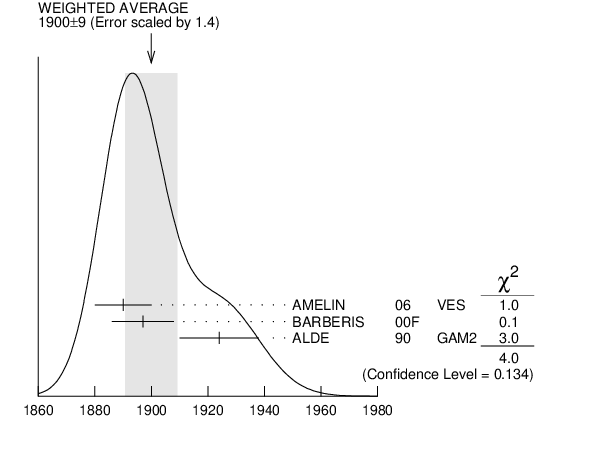# ${{\boldsymbol f}_{{2}}{(1910)}}$ ${{\boldsymbol \omega}}{{\boldsymbol \omega}}$ MODE INSPIRE search

VALUE (MeV) DOCUMENT ID TECN  COMMENT
$\bf{ 1900 \pm9}$ OUR AVERAGE  Error includes scale factor of 1.4.
$1890$ $\pm10$ 1
 2006
VES 36 ${{\mathit \pi}^{-}}$ ${{\mathit p}}$ $\rightarrow$ ${{\mathit \omega}}{{\mathit \omega}}{{\mathit n}}$
$1897$ $\pm11$
 2000 F
450 ${{\mathit p}}$ ${{\mathit p}}$ $\rightarrow$ ${{\mathit p}_{{f}}}{{\mathit \omega}}{{\mathit \omega}}{{\mathit p}_{{s}}}$
$1924$ $\pm14$
 1990
GAM2 38 ${{\mathit \pi}^{-}}$ ${{\mathit p}}$ $\rightarrow$ ${{\mathit \omega}}{{\mathit \omega}}{{\mathit n}}$
1  Supersedes BELADIDZE 1992B.${{\mathit f}_{{2}}{(1910)}}$ ${{\mathit \omega}}{{\mathit \omega}}$ MODE MASS (MeV)
References:
 AMELIN 2006
PAN 69 690 Resonances in the ${{\mathit \omega}}{{\mathit \omega}}$ System
 BARBERIS 2000F
PL B484 198 A Study of the ${{\mathit \omega}}{{\mathit \omega}}$ Channel Produced in Central ${{\mathit p}}{{\mathit p}}$ Interactions at 450 ${\mathrm {GeV/}}\mathit c$
 ALDE 1990
PL B241 600 Further Study of Mesons which Decay into ${{\mathit \omega}}{{\mathit \omega}}$